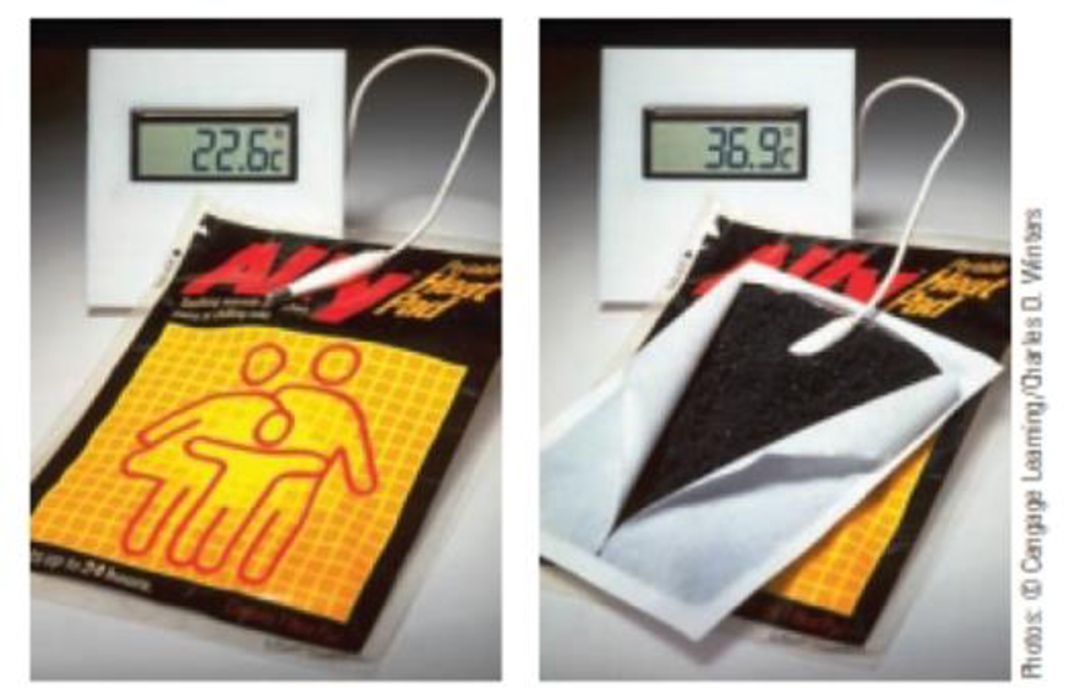Chapter 5, Problem 100IL

Chapter
Section
Textbook Problem

On a cold day, you can warm your hands with a “heat pad,” a device that uses the oxidation of iron to produce energy as heat.4 Fe(s) + 3 O2(g) → 2 Fe2O3(s)A hand warmer uses the oxidation of iron as a source of thermal energy.What mass of iron is needed to supply the energy required to warm 15 mL of water (d = 1.00 g/mL) from 23 °C to 37 °C?

Interpretation Introduction

Interpretation:

The required amount of Fe to warm 15mL of water has to be calculated.

Concept Introduction:

Standard enthalpy of the reaction,ΔrHo, is the change in enthalpy that happens when matter is transformed by a given chemical reaction, when all reactants and products are in their standard states. Enthalpy of the reaction,ΔrH, is the change in enthalpy that happens when matter is transformed by a given chemical reaction

Heat energy required to raise the temperature of 1g of substance by 1K..Energy gained or lost can be calculated using the below equation.

q=C×m×ΔT

Where, q= energy gained or lost for a given mass of substance (m), C =specific heat capacity,ΔT= change in temperature.

The standard enthalpy change of combustion of a compound is the enthalpy change which occurs when one gram of the compound is burned completely in oxygen under standard conditions, and with everything in its standard state.

ΔrH0=ΣnΔfH0(products)-ΣnΔfH0(reactants)

Explanation

Given,

ΔfH Of Fe=0

ΔfH Of oxygen = 0

ΔfH Of Fe2O3=-822.2kJ/mol

ΔrH0=ΣnΔfH0(products)-ΣnΔfH0(reactants)

ΔrH= (2mol×-822.2kJ/mol)- (0)

ΔrH=-1644kJ

Heat required to warm 15mL water

Specific capacity of heat of iron is 0

Still sussing out bartleby?

Check out a sample textbook solution.

See a sample solution

The Solution to Your Study Problems

Bartleby provides explanations to thousands of textbook problems written by our experts, many with advanced degrees!

Get Started